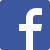Here is the latest from Julia Computing
BLG# Implementing Unums in Julia

29 Mar 2016 | Simon Byrne

This work was funded by the A*CRC and carried out in collaboration with Dr. John Gustafson and Dr. Marek Michalewicz. It was presented at the SuperComputing Frontiers 2016 in Singapore by Prof. Edelman.

The unum is a floating point format proposed by John Gustafson, proposed as an alternative to the now ubiquitious IEEE 754 formats. The proposal and justification are explained in his somewhat ambitiously-titled book The end of error, but the following slides give a good overview:

The two defining features of the unum format are:

• a variable-width storage format for both the significand and exponent, and
• an “u-bit”, which determines whether the unum corresponds to an exact number (u=0), or an interval between consecutive exact unums (u=1). In this way, the unums cover the entire extended real number line [-∞,+∞].

For performing computation with the format, Gustafson proposes using interval arithmetic with a pair of unums, what he calls an ubound, providing the guarantee that the resulting interval contains the exact solution (he also suggests a more complicated ubox method, but I won’t discuss this further).

# Unums.jl

The Unums.jl package provides an implementation of the unum format and ubound arithmetic in Julia.

New instances can be constructed from existing numbers

``````julia> x = Unum22(1)
Unums.Unum{2,2,UInt16}
1.0

julia> y = Unum22(2.1)
Unums.Unum{2,2,UInt16}
(2.0,2.125)
``````

As 2.1 (or, more correctly, the `Float64` nearest 2.1) cannot be represented as an exact Unum, the resulting Unum represents an open interval.

The low-level bit representation of an unum is similar to that of an IEEE754 floating point number, but with extra places for the ubit and the size of the exponent and fraction:

``````|sign|exponent|fraction|ubit|exponent size|fraction size|
``````

This is available via the `print_bits` function:

``````julia> Unums.print_bits(x)
|0|01|0|0|01|00|

julia> Unums.print_bits(y)
|0|1|0000|1|00|11|
``````

Standard arithmetic operations are defined, and Unums will automatically be promoted to Ubounds:

``````julia> x+y
Unums.Ubound{2,2,UInt16}
(3.0,3.125)

julia> x/y
Unums.Ubound{2,2,UInt16}
(0.9375,1.0)
``````

One of the Julia’s most powerful features are its generic methods. In particular, there are several linear algebra routines, such as LU and QR factorizations, which can be applied to an array of any numeric type, such as Quaternions, simply by defining the necessary elementary operations (`+`, `-`, `*`, `/` and `sqrt`) and a few simple methods such as `abs` and `copysign`.

We can make use of them here with Unums:

``````julia> X = map(Unum34,randn(10,6))
10x6 Array{Unums.Unum{3,4,UInt64},2}:
(0.76873779296875,0.7687454223632812)       …  (-1.520599365234375,-1.5205841064453125)
(1.505889892578125,1.5059051513671875)          (1.6515960693359375,1.651611328125)
(-0.21679306030273438,-0.21679115295410156)      (0.640228271484375,0.6402359008789062)
(0.3451271057128906,0.34513092041015625)       (-0.313323974609375,-0.3133201599121094)
(0.3553314208984375,0.3553352355957031)        (-1.01434326171875,-1.0143280029296875)
(2.5906982421875,2.590728759765625)         …  (-0.7313995361328125,-0.7313919067382812)
(0.5986404418945312,0.5986480712890625)         (0.007965564727783203,0.007965683937072754)
(0.4354515075683594,0.435455322265625)         (-1.214202880859375,-1.2141876220703125)
(-1.7028656005859375,-1.702850341796875)         (0.68011474609375,0.6801223754882812)
(-0.9994583129882812,-0.99945068359375)         (-1.211334228515625,-1.2113189697265625)

julia> Q,R = qr(X)
(
10x6 Array{Unums.Ubound{3,4,UInt64},2}:
(-0.203277587890625,-0.2031707763671875)      …   (0.5075531005859375,0.8198089599609375)
(-0.39812469482421875,-0.3980598449707031)       (-0.13979148864746094,0.17265892028808594)
(0.057305335998535156,0.057314395904541016)     (-0.3092536926269531,-0.1703510284423828)
(-0.09124469757080078,-0.09122943878173828)      (-0.062105655670166016,0.12235355377197266)
(-0.09394264221191406,-0.09392642974853516)       (0.016231060028076172,0.21294784545898438)
(-0.6849288940429688,-0.684814453125)         …  (-0.4050254821777344,-0.11310958862304688)
(-0.15826988220214844,-0.15824127197265625)      (-0.26529693603515625,-0.04947376251220703)
(-0.11512374877929688,-0.11510562896728516)       (0.2563362121582031,0.3899116516113281)
(0.4501228332519531,0.4501953125)               (-0.3648338317871094,-0.1708221435546875)
(0.264190673828125,0.2642326354980469)           (0.362701416015625,0.576568603515625)     ,

6x6 Array{Unums.Ubound{3,4,UInt64},2}:
(-3.782867431640625,-3.78271484375)  …   (0.4373779296875,0.4379119873046875)
0.0                                   (-1.2593994140625,-1.256591796875)
0.0                                    (0.31097412109375,0.31465911865234375)
0.0                                    (1.9207763671875,1.9372100830078125)
0.0                                   (-0.14617156982421875,-0.10152435302734375)
0.0                                …  (-2.2342529296875,-2.19671630859375)       )
``````

We can check that the matrix reconstructed from the factors contains the original matrix `X`:

``````julia> Y = Q*R
10x6 Array{Unums.Ubound{3,4,UInt64},2}:
(0.7685317993164062,0.7689743041992188)   …  (-1.89501953125,-1.15325927734375)
(1.5057373046875,1.5060577392578125)          (1.2778778076171875,2.025543212890625)
(-0.2168140411376953,-0.2167682647705078)      (0.4716682434082031,0.81317138671875)
(0.3450927734375,0.3451690673828125)         (-0.5455322265625,-0.08253669738769531)
(0.35529327392578125,0.3553733825683594)     (-1.248748779296875,-0.7854843139648438)
(2.5904541015625,2.59100341796875)        …  (-1.071014404296875,-0.38515472412109375)
(0.5985794067382812,0.5987167358398438)      (-0.2518424987792969,0.2725715637207031)
(0.4354095458984375,0.4355010986328125)      (-1.37896728515625,-1.0521697998046875)
(-1.703033447265625,-1.7026824951171875)       (0.4458961486816406,0.9188003540039062)
(-0.9995574951171875,-0.9993515014648438)     (-1.4760894775390625,-0.9517135620117188)

julia> all(map(in,X,Y))
true
``````

Unfortunately, as with any interval arithmetic approach, the problem is that the intervals grow as the number of operations increase. In this case, as each column of the orthogonal matrix `Q` depends on the previous ones, the widths increase with column number:

``````julia> W = map(Unums.width,Q)
10x6 Array{Float64,2}:
0.000106812  0.000665665  0.00137138   0.00675392  0.0597467  0.312256
6.48499e-5   0.000686646  0.00142288   0.0072937   0.0620613  0.31245
9.05991e-6   0.000112534  0.000808716  0.00331306  0.0285912  0.138903
1.52588e-5   0.000366211  0.000694275  0.00553513  0.0377731  0.184459
1.62125e-5   0.000196457  0.00105286   0.00414467  0.0401134  0.196717
0.000114441  0.000652313  0.00124741   0.00605774  0.0500031  0.291916
2.86102e-5   0.000366211  0.000835419  0.00431061  0.0451851  0.215823
1.81198e-5   0.000141144  0.000701904  0.00354385  0.0270338  0.133575
7.24792e-5   0.000396729  0.000806808  0.00379539  0.0399399  0.194012
4.19617e-5   0.000411987  0.000822067  0.00435543  0.0425873  0.213867

julia> spy(W, Scale.color_log10)
``````# Internals and performance

Internally, Unums.jl uses Julia’s `BigFloat` type (which wraps the MPFR library). This provides convenient per-operation rounding control and inexact flags, which are necessary for computing the correct intervals and determining the state of the u-bit. As a result, the performance is long way behind the corresponding machine floating point operations:

``````julia> X = ones(Ubound34,100,100);

julia> t = @elapsed X*X
14.853904448

julia> 2*100^3/t
134644.7331071454
``````

So that’s around 105 “unops” (unum operations per second), compared with

``````julia> peakflops()
5.945584096894352e10
``````

i.e. 6×1010 flops (floating point operations per second). Although it is not going to be possible to approach such speeds for unums without dedicated hardware support, it should be possible to see some gain via a lower-level implementation based on integer operations and bit-twiddling, as this would avoid many of the bottlenecks involved with the use of `BigFloat`s, such as memory allocation and garbage collection.

# Conclusions

I think the key lesson here is that although interval arithmetic is an extremely powerful tool, intervals cannot be used as simple substitutes for numbers. John Gustafson proposes using fused operations for polynomial evaluation and dot products, and it should be possible to test these ideas out in Julia, for example by overloading the `dot` function, or via macros to detect reused bindings in expressions such as `x*(2.0+x)`.

Fully leveraging the power of interval arithmetic does require a slightly different mindset, however, using specialised algorithms such as interval Newton’s method. Unfortunately, such approaches need to be designed from scratch, and as a result we can no longer fully leverage Julia’s generic functions.

I didn’t really discuss the utility of variable-width encoding. As it stands, the Unum format is far from the most efficient storage format, with a lot of redundant encodings. This problem that has been recognised by John Gustafson in his recent presentation on “Unums version 2.0”. Given the constraints of existing computing architectures, it may be that a byte-aligned format (similar to UTF-8) might be more amenable to optimization.

## Recent posts

CSV Reader Benchmarks: Julia Reads CSVs 10-20x Faster than Python and R
22 Jun 2020 | Deepak Suresh
JuliaTeam v2.0 Now Available
12 Jun 2020 | Julia Computing